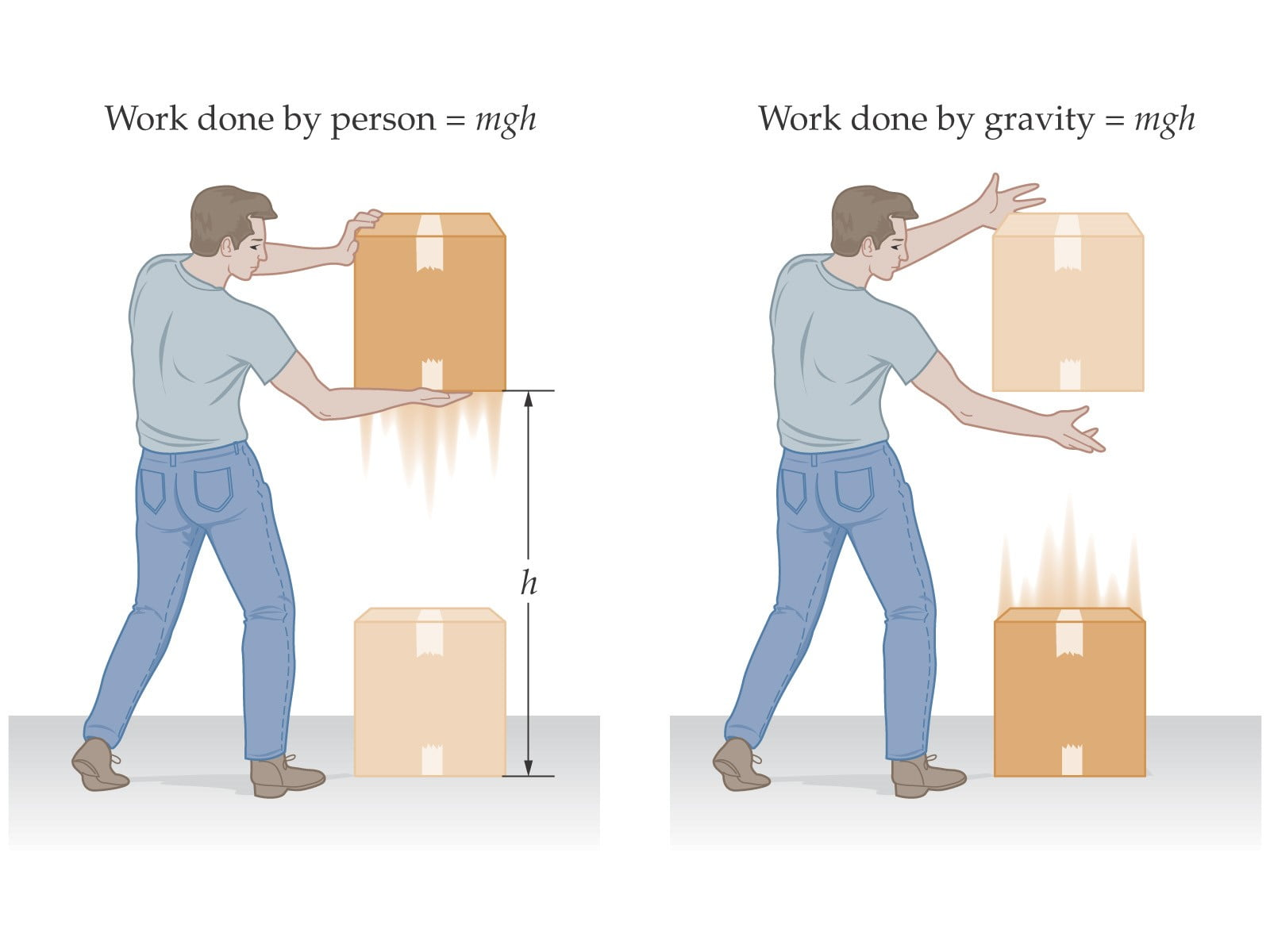# Conservative & Non-Conservative Forces & Gravitation

## Conservative And Non-Conservative Force

A conservative force is a kind of force supplies no energy body in a complete round trip. A conservative force does not do any work on any closed path.

Hence, when a body is thrown up, the kinetic energy of the body is decreased due to the downward pull of the earth. It reaches a maximum height and then starts coming back to earth again. During the downward portion, the downward pull of the earth, known as gravity, provides kinetic energy into the material. When it reaches the origin point, the active energy becomes the same as its initial kinetic energy.

The potential energy of the object was originally zero and becomes maximum when the body reaches its peak, and at the downfall, it becomes 0 again.

### What are some Conservative forces?

Gravitational and Spring forces are conservative force.

### What are Nonconservative Forces:

The work which cannot be recovered again after is called non-conservative force.

In short, What is done is done, and you cannot ctrl+z it. (you cannot recover it.) So how does this happen? Why can we not recover these forces?

Because this forces usually gets dissipated into heat.

### What are some non-conservative forces?

Friction and Forces exerted by muscles are nonconservative forces.

### Work Done by a Constant Force:

If the body moves towards the work done – the work is positive.
But if the body moves opposite to the work done – work is negative.

Example?
You are moving a box right – the box is moving right. Positive work!
The gravity is trying to push it down, but you have thrown a frisbee up, the frisbee is going up despite the gravity’s push down – It’s negative work!

## Kinetic Energy:

An object which has the motion is called Kinetic Energy. The motion can be either in the horizontal and vertical direction.

There are many types of Kinetic Motion. I am listing some of them:

1) Vibrational Kinetic Energy
2) Rotational Kinetic Energy
3) Translational Kinetic Energy

The formula of calculative K.E is 1/2 x m x v^2

Here m = object’s mass
v = velocity of the object

What is the kinetic energy of an object which has a mass of 10kg and velocity 5 m/s
Answer:

K.E = .5*10*5*5
=125 Joules

Facebook Comments